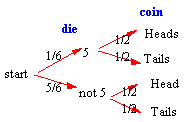### CFA Practice Question

There are 410 practice questions for this study session.

### CFA Practice Question

Roll a die and flip a coin, P(5 AND heads) = ______.

A. 1/2
B. 1/6
C. 1/12
Correct Answer: C

In a tree diagram, the event 5 AND heads is represented by the top branch. The probability of traveling this branch is (1/6)(1/2) = 1/12. Thus, P(5 and heads) = 1/12.### User Contributed Comments0

You need to log in first to add your comment.